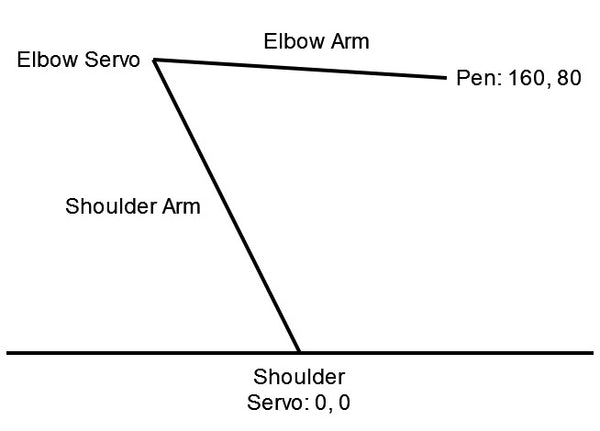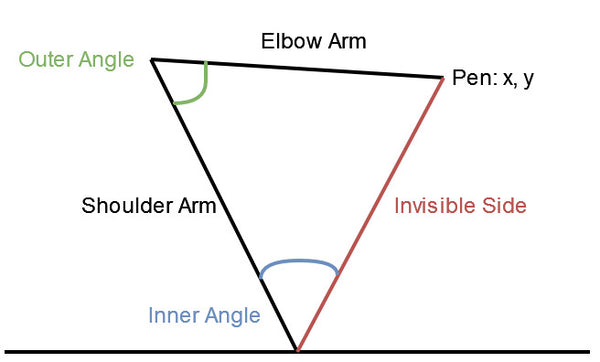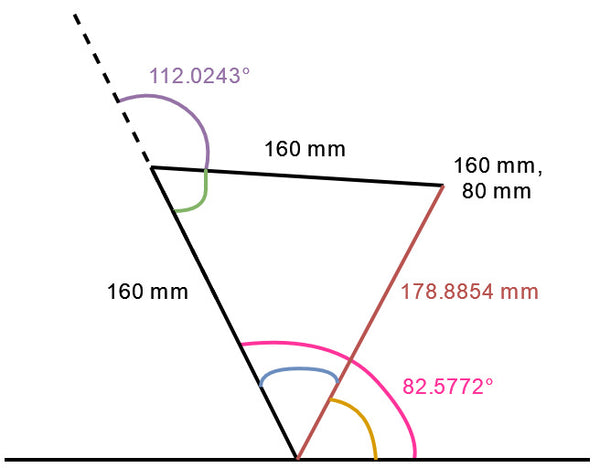# Overview

The biggest challenge behind making the Cardboard and Advanced drawing robot arms was figuring out how to move the arm to a specific position. This is needed so that we can use the line coordinates from our vectorised images to move the robot arm and draw the image. We need some way of converting the x, y coordinates into angles that we can use to set the shoulder servo and elbow servo.

The DrawingRobot library can be found on our GitHub repo.

The blogs in this series are:

# Pico Drawing Robot Arm Theory

Below is a diagram that shows the idea behind the drawing robot arm. We'll use the shoulder and elbow arm lengths from the CAD based drawing robot making them both 160 millimeters. We can imagine the shoulder servo is at point 0, 0 and we want our pen to be at 160, 80. The coordinates, like the arm lengths, are going to be in millimeters. To put the pen at 160, 80 the elbow servo will have to be at some x coordinate near the middle of the shoulder, but we don't actually need to know the exact coordinates of the elbow. We can't just tell the servos to move to these coordinates as they have no meaning to our servos. Instead we must calculate what angles the shoulder and elbow servos need to be at to position the pen at point 160, 80.We are going to be calculating the angles for our servos in radians before converting them to degrees. We will use radians for the calculations as the Python Math module returns values in radians for the angle related functions we are going to use. We then need to convert these angles from radians to degrees as our servo code sets the positions of the servos using angles in degrees.

Radians are a measurement of angles inside of a circle based on the radius of the circle. The measurement of the angle around half of a circle, or 180 degrees, in radians is PI. This is what allows us to calculate the circumference of a circle using the diameter times PI, as the diameter of a circle is 2 times its radius. To convert an angle from radians to degrees we multiply the angle by 180 and divide by PI.

To calculate our angles we need to start by imagining an invisible side between the pen and shoulder servo to create a triangle between our two servos and the pen. Then we need to calculate the length of the invisible side using Pythagoras Theorem with the x, y coordinates. For our example of moving the pen to 160, 80 this will give the invisible side a length of 178.8854. This is done by square rooting the sum of 1602 and 802:

√ x2 + y2Now that we have a triangle where we know the length of all three sides, we can use the law of cosines to calculate our inner and outer angles which will be used when setting the shoulder and elbow servos.

For the inner angle we know that the cosine of the inner angle is equal to:

(shoulderLength2 + invisibleLength2 - elbowLength2) / (2 * shoulderLength + inisibleLength)

This gives us 0.559017. When we then rearrange the formula to find the inner angle we find that the arc cosine of 0.559017 is equal to the inner angle in radians which is 0.9775965.

For the outer angle we perform the same set of calculations with different values. Instead we know the cosine of the outer angle is equal to:

(elbowLength2 + shoulderLength2 - invisibleLength2) / (2 * elbowLength + shoulderLength)

This gives us 0.375. When we then rearrange the formula to find the outer angle we find that the arc cosine of 0.375 is equal to the outer angle in radians which is 1.1864.To set the shoulder servo to the correct angle we also need to calculate the gap angle between the invisible side and our base. We can then add this gap angle to our inner angle to get full angle between the shoulder arm and base. To find the gap angle we convert the rectangular coordinates into polar coordinates using the inverse tangent function.

Polar coordinates are used to represent a coordinate using an angle from the base along with the distance between the base point and the coordinate. We can use polar coordinates to determine the angle between the invisible side and our base. We have already calculated the distance between our shoulder servo and the point we're moving our pen to using Pythagoras Theorem. Now we can find our gap angle which is equal to the arc tangent of 80 / 160 giving us an angle of 0.4636476 in radians.

atan(y / x)We have now collected all the information we need to calculate the angles our shoulder and elbow servos need to be positioned at to move the pen to 160, 80. For our shoulder servo we can find the angle it needs to be at by adding the inner angle to the gap angle giving us 1.4412441 in radians. Then we can convert this to degrees which gives us a shoulder servo angle of 82.5772. For our elbow servo we can find the angle it needs to be at by minusing the outer angle from PI giving us 1.9551 in radians. We use PI here to calculate our angle backwards from 180 degrees. Setting our elbow servo to 180 degrees positions it to face back at the shoulder servo and the calculation we did for our outer angle is from the shoulder to the pen. We can again convert our angle to degrees which gives us an elbow servo angle of 112.0243.# Pico Drawing Robot Arm Code

Now let's see how we can use these idea to convert an x, y coordinate to the angles for our servos. We define a function called plot that takes the two x, y coordinate values as its inputs. We then offset these x and y values using variables we setup earlier in our DrawingRobot library. These offsets stop the robot arm from drawing too close to the base of the arm. Then using the x and y values we apply Pythagoras Theorem to calculate the length of our invisible side and store it in the lenHypot variable. Next we want to check if the length of the invisible side is longer than the length of our two arms, as this would be out of reach of our robot.

``````def plot(self, x, y):
x += self.xOffset
y += self.yOffset
lenHypot = sqrt(x ** 2 + y ** 2)

if lenHypot > self.shoulderLength + self.elbowLength:
return 0, 0
``````

Our next step is to use the law of cosines to calculate our inner and outer angles using the three side lengths of our triangle. This uses the math module's acos function which is used to find the arc cosine of a number.

``````    angleInner = acos((self.shoulderLength ** 2 + lenHypot ** 2 - self.elbowLength ** 2)
/ (2 * self.shoulderLength * lenHypot))
angleOuter = acos((self.elbowLength ** 2 + self.shoulderLength ** 2 - lenHypot ** 2)
/ (2 * self.elbowLength * self.shoulderLength))
``````

With the two angles inside of our triangle calculated, we need to find the gap angle using polar coordinates. This is done using the math module's arc tangent of y over x, returning the angle in radians.

``````    angleGap = atan2(y, x)
``````

Finally, we convert our angles into degrees for both the shoulder angle and elbow angle before returning these angles. We can then use the returned angles to gradually move the shoulder and elbow servos into these positions. When they reach these positions the pen will be at the coordinates x and y.

``````    shoulderAngle = degrees(angleInner + angleGap)
elbowAngle = degrees(pi - angleOuter)

return shoulderAngle, elbowAngle
``````

Below is the full code snippet for converting the x, y coordinates to the angles used by the shoulder and elbow servos.

``````# Convert an x, y coordinate into shoulder and elbow servo angles
def plot(self, x, y):
# Offset the x and y coordinates
x += self.xOffset
y += self.yOffset
# Calculate the length of the invisible side
lenHypot = sqrt(x ** 2 + y ** 2)

# Stop if the coordinate is too far to reach
if lenHypot > self.shoulderLength + self.elbowLength:
return 0, 0

# Calculate the angles inside the triangle for the shoulder and elbow
angleInner = acos((self.shoulderLength ** 2 + lenHypot ** 2 - self.elbowLength ** 2)
/ (2 * self.shoulderLength * lenHypot))
angleOuter = acos((self.elbowLength ** 2 + self.shoulderLength ** 2 - lenHypot ** 2)
/ (2 * self.elbowLength * self.shoulderLength))
# Calculate the angle of the gap between the base and start of the triangle
angleGap = atan2(y, x)

# Convert the angles from radians to degrees
shoulderAngle = degrees(angleInner + angleGap)
elbowAngle = degrees(pi - angleOuter)

return shoulderAngle, elbowAngle
``````

With the plot function complete we can then use it to move our pen to an x, y coordinate. We could just tell our servos to move the the angles returned by plot but this would cause our robot arm to jolt into position and wouldn't create a very precise line. Instead we use the moveTo function, full code on our GitHub, to gradually move the servos to the positions we need in small steps.

We do this by calculating the difference between the current servo angles and the desired servo angles. Using this, we then divide the angle differences by the maximum difference times 4 to create the steps we'll use to move the servos. This makes sure both servos make little adjustments to their angles over time to move to their new positions. With our steps defined, the moveTo function then continuously moves the servos by our steps until the servos are less than one step away from their desired position. At this point, the difference between the angles are so small that we just set the servo angles to the new position, completing the move of the pen to the x, y coordinate.

With our moveTo function also complete, the rest of our DrawingRobot functions now make use of moveTo to move the robot arm and draw on our paper. The moveTo function is used in conjunction with the penUp and penDown functions to lift the pen up from the paper which stops it from drawing, and push the pen down on to the paper which allows it to draw. The drawImage function simply retrieves the line coordinates from the text file and moves the pen to each of the coordinates on the line. It then makes use of the penUp and penDown functions to lift the pen up between drawing lines and push the pen down when drawing a line.